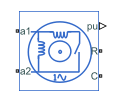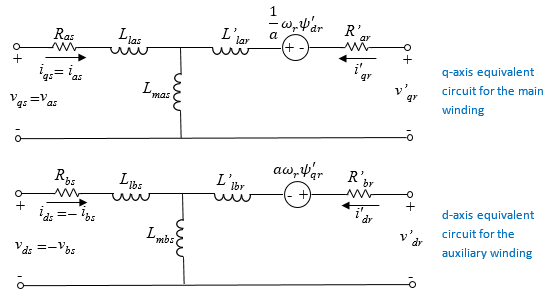# Induction Machine (Single-Phase)

Single-phase induction machine with SI or pu fundamental parameterization

•Libraries:
Simscape / Electrical / Electromechanical / Asynchronous

## Description

The Induction Machine (Single-Phase) block represents a single-phase induction machine with a squirrel cage rotor with fundamental parameters expressed in per-unit or in the International System of Units (SI).

This block models four types of single-phase induction machines:

• `Split-phase`

• `Capacitor-start`

• `Capacitor-start-capacitor-run`

• `Main and auxiliary windings`

The single phase induction machine consists of a squirrel cage rotor and two stator windings, the main and the auxiliary windings. The auxiliary winding is typically only active during startup. However, to improve performance, the auxiliary winding can be active during running for low-power applications. The figure shows the equivalent d- and q-axis circuits for the main and auxiliary windings.The table defines the variables.

VariableDefinition
vqsvdsStator voltages in the d-q representation
iqsidsStator currents in the d-q representation
ΨqrΨdrRotor fluxes in the d-q representation
Rotor electrical speed
aAuxiliary/main windings turn ratio
RasMain winding stator resistance
LasMain winding stator leakage inductance
R'arMain winding rotor resistance
L'larMain winding rotor leakage inductance
LmasMain winding mutual inductance
RbsAuxiliary winding stator resistance
RlbsAuxiliary winding stator leakage inductance
R'brAuxiliary winding rotor resistance
L'lbrAuxiliary winding rotor leakage inductance
LmbsAuxiliary winding mutual inductance

### Equations

The SI model converts the SI values that you enter in the dialog box to per-unit values for simulation. For information on the relationship between SI and per-unit machine parameters, see Per-Unit Conversion for Machine Parameters. For information on per-unit parameterization, see Per-Unit System of Units.

These transformations reduce the rotor resistance, rotor leakage inductance, and stator mutual inductance to a single set of values by defining the equivalent circuits with respect to the main winding:

`${L}_{ms}={L}_{mas}=\frac{1}{{a}^{2}}{L}_{mbs}$`

`${R}_{ar}^{\text{'}}=\frac{1}{{a}^{2}}{R}_{br}^{\text{'}}$`

`${L}_{lar}^{\text{'}}=\frac{1}{{a}^{2}}{L}_{lbr}^{\text{'}}$`

The voltage equations for the stator and rotor are:

`${v}_{qs}={R}_{as}{i}_{qs}+\frac{d{\psi }_{qs}}{dt}$`

`${v}_{ds}={R}_{bs}{i}_{ds}+\frac{d{\psi }_{ds}}{dt}$`

`$0={R}_{ar}^{\text{'}}{i}_{qr}^{\text{'}}+\frac{d{\psi }_{qr}^{\text{'}}}{dt}-\frac{1}{a}{\omega }_{r}{\psi }_{dr}^{\text{'}}$`

`$0={a}^{2}{R}_{ar}^{\text{'}}{i}_{dr}^{\text{'}}+\frac{d{\psi }_{dr}^{\text{'}}}{dt}+a{\omega }_{r}{\psi }_{qr}^{\text{'}}$`

`${\psi }_{qs}=\left({L}_{las}+{L}_{ms}\right){i}_{qs}+{L}_{ms}{i}_{qr}^{\text{'}}$`

`${\psi }_{ds}=\left({L}_{lbs}+{a}^{2}{L}_{ms}\right){i}_{ds}+{a}^{2}{L}_{ms}{i}_{dr}^{\text{'}}$`

`${\psi }_{qr}^{\text{'}}=\left({L}_{lar}^{\text{'}}+{L}_{ms}\right){i}_{qr}^{\text{'}}+{L}_{ms}{i}_{qs}$`

`${\psi }_{dr}^{\text{'}}={a}^{2}\left({L}_{lar}^{\text{'}}+{L}_{ms}\right){i}_{dr}^{\text{'}}+{a}^{2}{L}_{ms}{i}_{ds}$`

The expression for the electromagnetic torque, T, is obtained by applying the principle of virtual displacement .

`$T=p\left(a{\psi }_{qr}^{\text{'}}{i}_{dr}^{\text{'}}-\frac{1}{a}{\psi }_{dr}^{\text{'}}{i}_{qr}^{\text{'}}\right)$`

The mechanical equation is

`$J\frac{d{\omega }_{m}}{dt}=T-{T}_{L}-{B}_{m}{\omega }_{m},$`

where ωm is the rotor angular velocity.

In split-phase machines, the auxiliary winding is displaced at 90 electrical degrees from the main winding and operates only until the speed reaches the disconnection speed, which is typically 70 to 80 percent of rated speed. In this configuration, the auxiliary winding has high resistance and small reactance compared to the main winding. The resulting phase difference makes the machine behave like a two-phase machine.

The capacitor-start machine is a type of split-phase machine that uses a capacitor in series with the auxiliary winding to start the machine. In this configuration, auxiliary and main windings have the same number of turns. The value of the capacitor ensures that the current in the auxiliary coil leads the current in the main winding by approximately 80 electrical degrees.

The capacitor-start voltage is:

`${v}_{c}={R}_{s}{i}_{ds}+{\int }^{\text{​}}\frac{1}{{C}_{s}}{\omega }_{r}{i}_{ds}.$`

When a capacitor is connected in series with the auxiliary winding, the voltage equation for the d-axis is

`${v}_{ds}={R}_{bs}{i}_{ds}+\frac{d{\psi }_{ds}}{dt}-{R}_{s}{i}_{ds}-{\int }^{\text{​}}\frac{1}{{C}_{s}}{\omega }_{r}{i}_{ds}.$`

The extension is obtained immediately for capacitor-start-capacitor-run machines. In this configuration, two capacitors are connected in parallel.The d-axis voltage after disconnecting the capacitor-start is

`${v}_{ds}={R}_{bs}{i}_{ds}+\frac{d{\psi }_{ds}}{dt}-{R}_{r}{i}_{ds}-{\int }^{\text{​}}\frac{1}{{C}_{r}}{\omega }_{r}{i}_{ds}.$`

### Display Option

To display the machine per-unit base values in the MATLAB® Command Window, right-click the block and, from the Electrical menu, select Display Base Values.

### Model Thermal Effects

You can expose thermal ports to simulate the effects of generated heat and motor temperature. To expose the thermal ports and the Thermal parameters, set the Modeling option parameter to either:

• `No thermal port` — The block contains electrical conserving ports associated with the stator windings, but does not contain thermal ports.

• `Show thermal port` — The block contains expanded electrical conserving ports associated with the stator windings and thermal conserving ports for each of the windings and for the rotor.

For more information about using thermal ports in actuator blocks, see Simulating Thermal Effects in Rotational and Translational Actuators.

### Variables

To set the priority and initial target values for the block variables before simulation, use the Initial Targets section in the block dialog box or Property Inspector. For more information, see Set Priority and Initial Target for Block Variables.

Nominal values provide a way to specify the expected magnitude of a variable in a model. Using system scaling based on nominal values increases the simulation robustness. You can specify nominal values using different sources, including the Nominal Values section in the block dialog box or Property Inspector. For more information, see System Scaling by Nominal Values.

## Ports

### Output

expand all

Physical signal vector port associated with the machine per-unit measurements. The vector elements are:

• pu_torque

• pu_velocity

• pu_vds

• pu_vqs

• pu_v0s = 0

• pu_ids

• pu_iqs

• pu_i0s = 0

### Conserving

expand all

Electrical conserving port associated with the main winding positive terminal.

Electrical conserving port associated with the main winding negative terminal.

Electrical conserving port associated with the auxiliary winding positive terminal.

#### Dependencies

This port is visible only if you set the Type to ```Main and auxiliary windings```.

Electrical conserving port associated with the auxiliary winding negative terminal.

#### Dependencies

This port is visible only if you set the Type to ```Main and auxiliary windings```.

Mechanical rotational conserving port associated with the machine rotor.

Mechanical rotational conserving port associated with the machine case.

Thermal conserving port associated with stator main winding.

#### Dependencies

To enable this port, set Modeling option to ```Show thermal port```.

Thermal conserving port associated with stator auxiliary winding.

#### Dependencies

To enable this port, set Modeling option to `Show thermal port`.

Thermal conserving port associated with the rotor.

#### Dependencies

To enable this port, set Modeling option to `Show thermal port`.

## Parameters

expand all

Whether to enable the thermal ports of the block and model the effects of generated heat and motor temperature.

### Main

Type of induction machine.

Rated apparent power of the induction machine.

RMS voltage.

Nominal electrical frequency corresponding to the rated apparent power.

Number of machine pole pairs. The value is used as a back-electromotive force constant.

Unit system for block parameterization.

#### Dependencies

Selecting:

• `SI` exposes SI Electrical parameters.

• `Per unit` exposes per-unit Electrical parameters.

### Electrical

For the Parameterization unit parameter in the Main settings, select `SI` to expose the SI Electrical parameters, or ```Per unit``` to expose the per-unit Electrical parameters.

SI stator resistance for the main winding.

#### Dependencies

Selecting `SI` for the Parameterization unit parameter in the Main settings exposes SI Electrical parameters.

SI leakage inductance for the main winding.

#### Dependencies

Selecting `SI` for the Parameterization unit parameter in the Main settings exposes SI Electrical parameters.

SI rotor resistance for the main winding.

#### Dependencies

Selecting `SI` for the Parameterization unit parameter in the Main settings exposes SI Electrical parameters.

SI rotor leakage inductance for the main winding.

#### Dependencies

Selecting `SI` for the Parameterization unit parameter in the Main settings exposes SI Electrical parameters.

SI mutual inductance for the main winding.

#### Dependencies

Selecting `SI` for the Parameterization unit parameter in the Main settings exposes SI Electrical parameters.

SI stator resistance for the auxiliary winding.

#### Dependencies

Selecting `SI` for the Parameterization unit parameter in the Main settings exposes SI Electrical parameters.

SI stator leakage inductance for the auxiliary winding.

#### Dependencies

Selecting `SI` for the Parameterization unit parameter in the Main settings exposes SI Electrical parameters.

Winding ratio between the main and auxiliary winding.

Per-unit stator resistance for the main winding.

#### Dependencies

Selecting `Per unit` for the Parameterization unit parameter in the Main settings exposes per-unit Electrical parameters.

Per-unit leakage inductance for the main winding.

#### Dependencies

Selecting `Per unit` for the Parameterization unit parameter in the Main settings exposes per-unit Electrical parameters.

Per-unit rotor resistance for the main winding.

#### Dependencies

Selecting `Per unit` for the Parameterization unit parameter in the Main settings exposes per-unit Electrical parameters.

Per-unit rotor leakage inductance for the main winding.

#### Dependencies

Selecting `Per unit` for the Parameterization unit parameter in the Main settings exposes per-unit Electrical parameters.

Per-unit mutual inductance for the main winding.

#### Dependencies

Selecting `Per unit` for the Parameterization unit parameter in the Main settings exposes per-unit Electrical parameters.

Per-unit stator resistance for the auxiliary winding.

#### Dependencies

Selecting `Per unit` for the Parameterization unit parameter in the Main settings exposes per-unit Electrical parameters.

Per-unit stator leakage inductance for the auxiliary winding.

#### Dependencies

Selecting `Per unit` for the Parameterization unit parameter in the Main settings exposes per-unit Electrical parameters.

Winding ratio between the main and auxiliary winding.

SI capacitor-start resistance.

#### Dependencies

To enable this parameter, set Type to either `Capacitor-start-capacitor-run` or `Capacitor-start` and Parameterization unit to `SI`.

SI capacitor-start capacitance.

#### Dependencies

To enable this parameter, set Type to either `Capacitor-start-capacitor-run` or `Capacitor-start` and Parameterization unit to `SI`.

SI capacitor-run resistance.

#### Dependencies

To enable this parameter, set Type to `Capacitor-start-capacitor-run` and Parameterization unit to `SI`.

SI capacitor-run capacitance.

#### Dependencies

To enable this parameter, set Type to `Capacitor-start-capacitor-run` and Parameterization unit to `SI`.

Per-unit capacitor-start resistance.

#### Dependencies

To enable this parameter, set Type to `Capacitor-start-capacitor-run` and Parameterization unit to ```Per unit```.

Per-unit capacitor-start capacitance.

#### Dependencies

To enable this parameter, set Type to `Capacitor-start-capacitor-run` and Parameterization unit to ```Per unit```.

Per-unit capacitor-run resistance.

#### Dependencies

To enable this parameter, set Type to `Capacitor-start-capacitor-run` and Parameterization unit to ```Per unit```.

Per-unit capacitor-run capacitance.

#### Dependencies

To enable this parameter, set Type to `Capacitor-start-capacitor-run` and Parameterization unit to ```Per unit```.

Speed when capacitor is disconnected, expressed as a percentage of rated speed. The value must be between `0` and `100`, inclusive.

#### Dependencies

To enable this parameter, set Type to either `Split-phase`, `Capacitor-start`, or `Capacitor-start-capacitor-run`.

### Mechanical

Inertia of the rotor.

Damping of the rotor.

### Thermal

These parameters appear only for blocks with exposed thermal ports.

Temperature for which motor parameters are quoted.

Coefficient α in the equation relating resistance to temperature for all three windings, as described in Thermal Model for Actuator Blocks. The default value, `3.93e-3` 1/K, is for copper.

Coefficient relating cage resistance to temperature for the rotor. The default value, `4.4e-3` 1/K, is for aluminium.

Thermal mass value for each stator winding. The thermal mass is the energy required to raise the temperature by one degree.

Thermal mass of the rotor. The thermal mass is the energy required to raise the temperature of the rotor by one degree.

 Krause, P. C. "Simulation of Unsymmetrical 2-Phase Induction Machines." IEEE Transactions on Power Apparatus and Systems. Vol 84, Number 11, 1965, pp. 1025-1037.